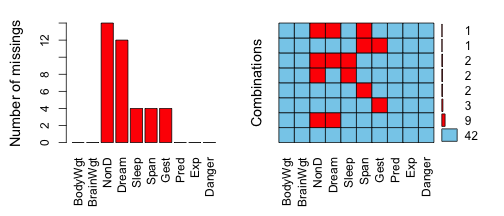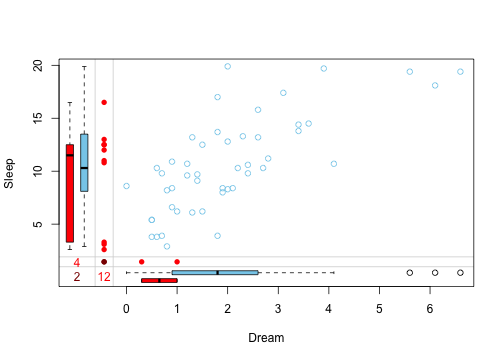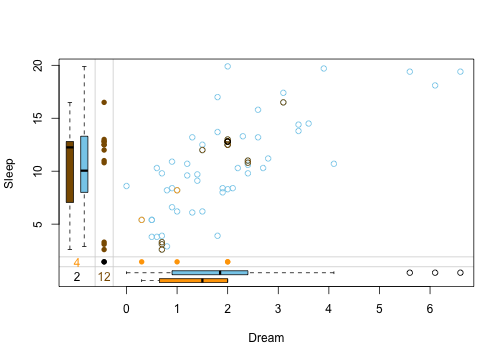VIM introduces tools for visualization of missing and imputed values. Forthermore, methods to impute missing values are featured. This vignette will give a brief look at a common imputation scenario and showcase how VIM can be used to both impute the data and also interpret the results visually.

### Visualize missing values

``````library(VIM)
data(sleep)
a <- aggr(sleep, plot = FALSE)
plot(a, numbers = TRUE, prop = FALSE)
``````The left plot shows the amount of missings for each column in the dataset `sleep` and the right plot shows how often each combination of missings occur. For example, there are 9 rows wich contain a missing in both `NonD` and `Dream`.

For simplicity, we will only look at the variables `Dream` and `Sleep` for the remainer of this vignette. Bivariate datasets can be passed to special functions that visualize the structure of missings such as `marginplot()`.

``````x <- sleep[, c("Dream", "Sleep")]
marginplot(x)
``````The red boxplot on the left shows the distrubution of all values of `Sleep` where `Dream` contains a missing value. The blue boxplot on the left shows the distribution of the values of `Sleep` where `Dream` is observed.

### Impute missing values

In order to impute missing values, `VIM` offers a spectrum of imputation methods like `kNN()` (k nearest neighbor), `hotdeck()` and so forth. Those functions can be applied to a `data.frame` and return another `data.frame` where missings are replaced by imputed values.

``````x_imputed <- kNN(x)
``````

Note however, that in certain circumstances, there will be missings left in the returned dataset depending on the method used.

### Visualize imputed values

The same functions that visualize missing values can also visualize the imputed dataset.

``````marginplot(x_imputed, delimiter = "_imp")
``````In this plot three differnt colors are used in the top-right. These colors represent the structure of missings.

• brown points represent values where `Dream` was missing initially
• beige points represent values where `Sleep` was missing initially
• black points represent values where both `Dream` and `Sleep` were missing initially

The `kNN()` method seemingly preserves the correlation between `Dream` and `Sleep`.